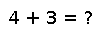# with_bigfloat_precision

with_bigfloat_precision(f::Function,precision::Integer)

Change the `BigFloat` arithmetic precision (in bits) for the duration of `f`. It is logically equivalent to:

``````old = get_bigfloat_precision()
set_bigfloat_precision(precision)
f()
set_bigfloat_precision(old)``````

## Examples

Checking you are not a robot: